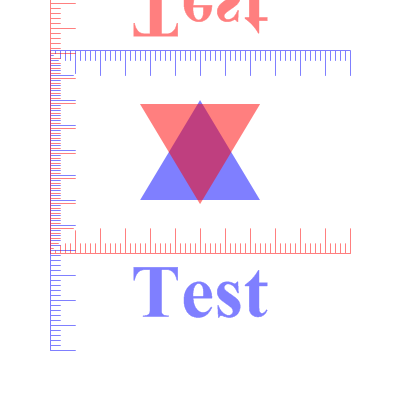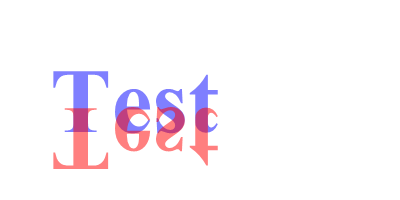FlipCoordinatesY()

Syntax

FlipCoordinatesY(AxisY.d [, System])
Description
Mirrors the vector drawing coordinate system at the specified Y axis.

Parameters

 AxisY.d The Y coordinate at which the coordinate system should be mirrored. System (optional) Specifies the coordinate system to change. This can be one of the following values: ``` #PB_Coordinate_User : Change the coordinate system for points in the drawing path (default) #PB_Coordinate_Source: Change the coordinate system for the vector drawing source ```

Return value

None.

Remarks

See the vectordrawing overview for an introduction to the different coordinate systems. The following image demonstrates the effect of flipped coordinates. The same figure is drawn twice, the original is in blue, and the version with flipped coordinates is in red.Example

```  If OpenWindow(0, 0, 0, 400, 200, "VectorDrawing", #PB_Window_SystemMenu | #PB_Window_ScreenCentered)
CanvasGadget(0, 0, 0, 400, 200)

If StartVectorDrawing(CanvasVectorOutput(0))
VectorFont(LoadFont(0, "Times New Roman", 60, #PB_Font_Bold))

VectorSourceColor(RGBA(0, 0, 255, 128))
MovePathCursor(50, 50)
DrawVectorText("Test")

FlipCoordinatesY(120)

VectorSourceColor(RGBA(255, 0, 0, 128))
MovePathCursor(50, 50)
DrawVectorText("Test")

StopVectorDrawing()
EndIf

Repeat
Event = WaitWindowEvent()
Until Event = #PB_Event_CloseWindow
EndIf
```See Also

ResetCoordinates(), TranslateCoordinates(), ScaleCoordinates(), RotateCoordinates(), SkewCoordinates(), FlipCoordinatesX(), ConvertCoordinateX(), ConvertCoordinateY()

Supported OS

All

<- FlipCoordinatesX() - VectorDrawing Index - IsInsidePath() ->